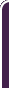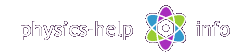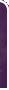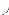homephysics online guidephysics help my info terms of use contactsWhat is Physics? Mechanics Electricity and Magnetism Electric Field Gauss' Law about below subjects Electric Potential Capacity Direct Current Magnetic Field Magnetic Field Laws Magnetic Interactions Electromagnetic Induction Maxwell's Equations Oscillations and Waves Simple Harmonic Motion Damped Harmonic Motion Driven Harmonic Motion Electric Oscillation Alternating Current Wave Motion Elastic Waves Electromagnetic Waves Optics Light Waves Geometrical Optics Interference Polarization Diffraction Fraunhofer Diffraction Dispersion, Absorption, Diffusion Doppler Effect Thermodynamics Ideal Gas Molecular Statistics Transport Phenomena First Law of Thermodynamics Second and Third Laws of Thermodynamics Imperfect Gas Liquids Solids Quantum Physics Thermal Radiation Quantum Properties of Light Wave Properties of Particles Planetary Model of Atom X-Rays Particle in Potential Well Pauli Exclusion Principle Nuclear Physics Solid State Physics Appendices# Gravitation

Gravitation investigates motion of objects under the force of gravityHere (all units see here):

M and m are masses of objectsis gravitational force on m from M

-is gravitational force on M from mis position vector of m with respect to Mis velocity vector of mis total acceleration vector of m

h is height of m above M

## General formulas

Gravitational force vector is defined by Newton's law of gravitationwhere G is universal gravitational constant

Magnitude of gravitational forceWeight of object near the surface of the earth at height hwhere:

m is mass of the object

Weight of object on the surface on the earthFree-fall acceleration vectorMagnitude of free-fall acceleration near the surface of the earthMagnitude of free-fall acceleration on the surface of the earthRelation between weigh and massGravitational potential energy with respect to infinitely far pointGravitational potential energy of object in the vicinity of the surface at height h## Motion of object around the earthThe total linear acceleration of the objectPrinciple of conservation of energy for the objectLaw of conservation of angular momentum of the objectSpeed required for object to become artificial satellite near the surfaceSpeed required for object to escape earth's gravity from the surface## Kepler's laws for motion of planets around the sunWhere d is length of major axis of elliptical orbit

Kepler's first law: all planets are moving around the sun in elliptical orbits with the sun at one of the focuses

Kepler's second law: for any planet the line joining the planet to the sun sweeps out the same amount of area in a given moment of time no matter where the plane is on its orbit. Which is defined by vector product equationKepler's third law: the ratio of period of orbital motion of planet squared to length of major axis of its orbit cubed is the same for all planetswhere T is period of orbital motionhome  |  physics online guide  |  physics help  |  my info  |  terms of use  |  contacts Dr. Nikitin © 2010